## Failures

Firstly I use IDA Pro to open it, being informed that it is AMD64 and compressed by UPX. So I download `UPX394w` to uncompress it. Then in IDA Pro you can follow XREF of the string “Who Are You?” and find the main logic stream: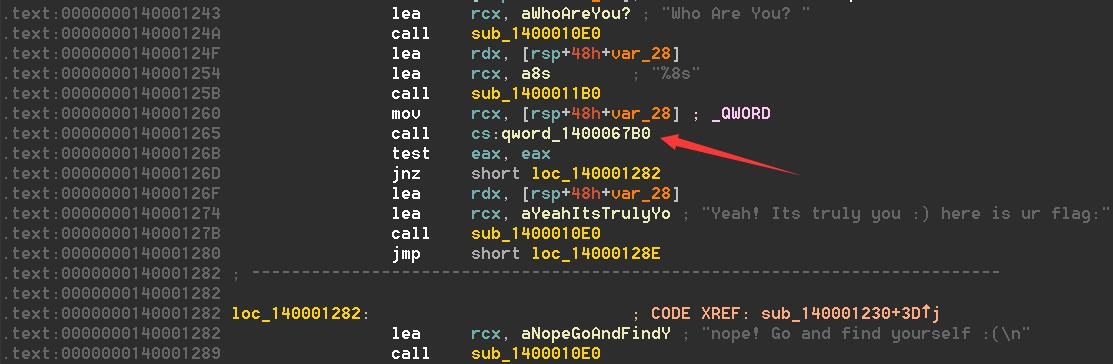Notice that

``````call    cs:qword_1400067B0
``````

But if you go to `0x1400067B0` you may probably see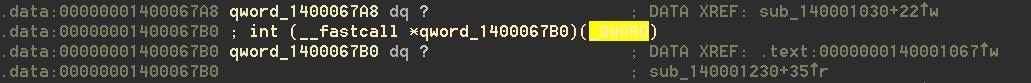Now it’s time to do dynamic analysis to see what’s going on. So I use x64dbg and follow it til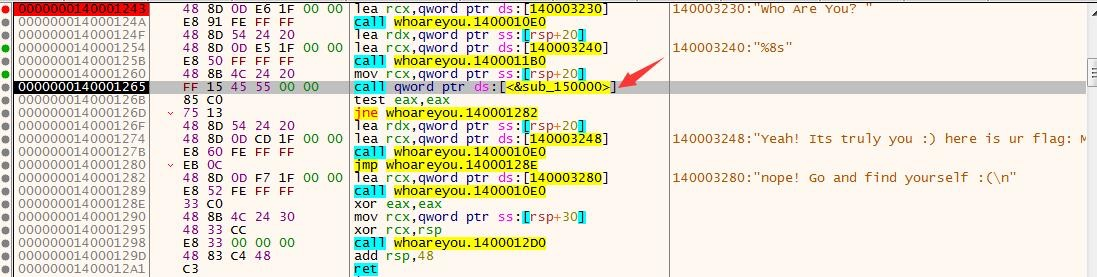And `F7` to step in then you will see the encryption algorithm: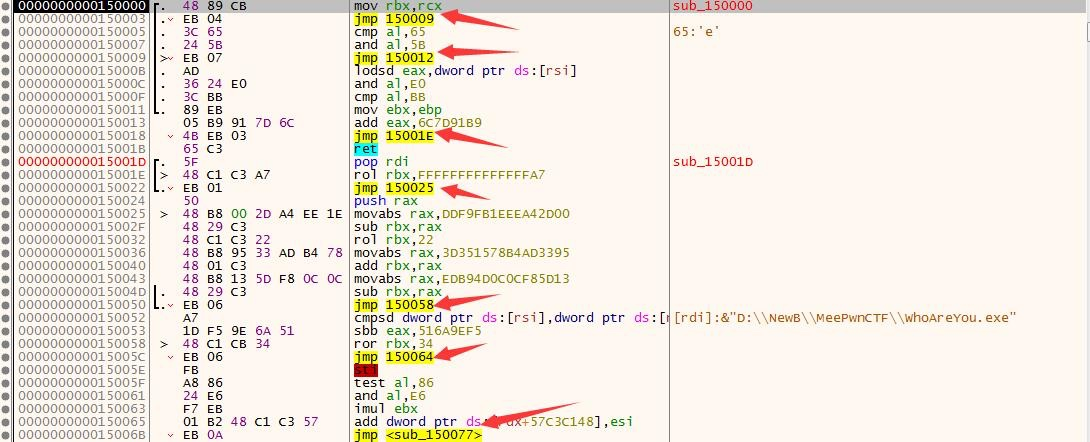It seems that the encryption algorithm is chained by lots of `jmp` instructions. So what to do next? The flag’s length is 8 so brute force in the dictionary of `[a-zA-Z0-9]` may be possible (if you are enough lucky) but anyway it is not a good idea.

So I plan to extract the encryption algorithm instructions and manage to write out the decryption algorithm. I don’t know how to extract instructions from x64dbg, so I plan to find them in IDA Pro and copy them out.

First, I search in HxD for one specific instruction and follow the file offset I get from HxD in IDA Pro. And I find them: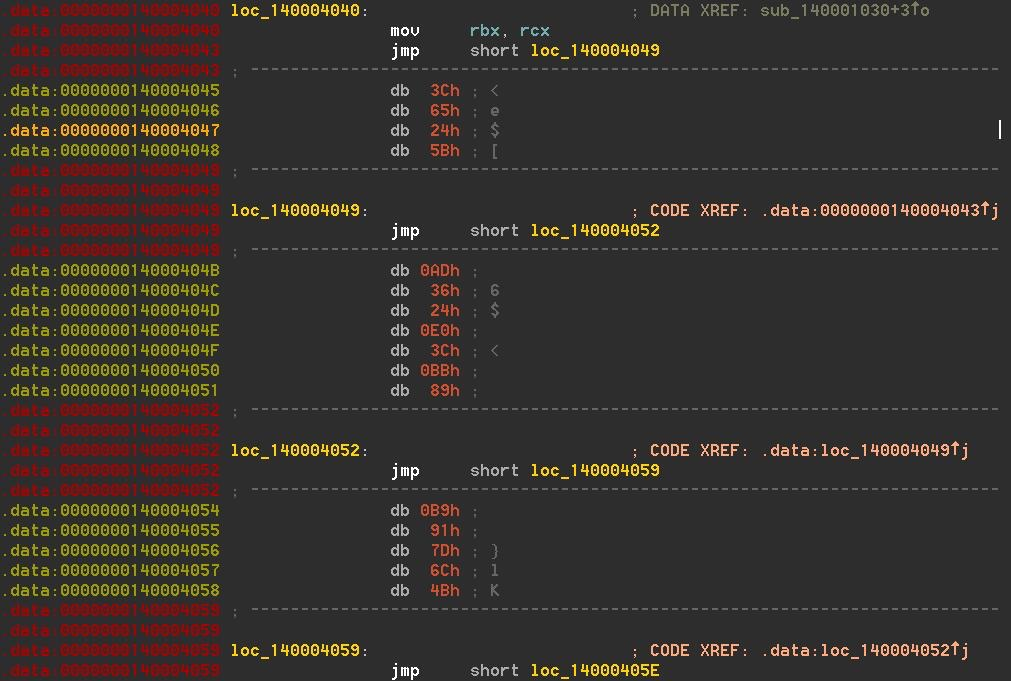I copy all of them into Sublime Text and apply Regex replacing function on them. Finally the instruction batch is like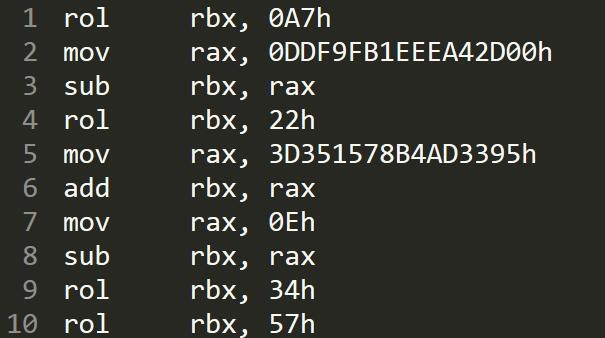Pay attention to your regular expression, case sensitive option and so on.

Tips:

`\s+\$` stands for one empty line.

Now look at the encryption algorithm and it is easy to write out the decryption algorithm:

First, use `tac` on Linux to fetch the inverse text (inverse.asm).

Second, I write `decryption.py` to generate decryption assembly language code (ans.asm):

``````f = open("inverse.asm")
f.close()
ff = open("ans.asm", "w")

w = z.split("\n")
l = len(w)

for i in range(l):
if w[i] == '':
continue
if w[i][:3] == 'sub':
ff.write(w[i+1] + '\n')
ff.write(s + '\n')
s = "sub" + w[i][3:]
ff.write(w[i+1] + '\n')
ff.write(s + '\n')
elif w[i][:3] == 'rol':
s = 'ror' + w[i][3:]
ff.write(s + '\n')
elif w[i][:3] == 'ror':
s = 'rol' + w[i][3:]
ff.write(s + '\n')
elif w[i][:3] == 'xor':
ff.write(w[i+1] + '\n')
ff.write(w[i] + '\n')
elif w[i][:3] == 'mov':
continue
else:
print("unknown: " + w[i])

ff.close()
``````

Then plug ans.asm into the location of `{Content}` in run.asm below:

``````[section .text]

global main

main:

push rbp
mov rbp, rsp

{Content}

mov rsp, rbp
pop rbp

ret
``````

And compile on Linux:

``````nasm -f elf64 run.asm
gcc -o run run.o
``````

Then use GDB and make breakpoint at last and use `p/x \$rbx` to see the flag.

What I get is `f4k3f4k3`. But it is not the correct flag (just a fake).

Input it in the uncompressed binary, it seems correct: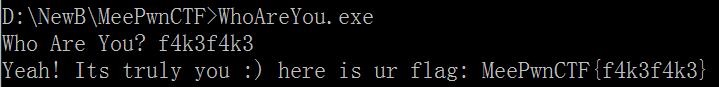But in the original binary, it is wrong: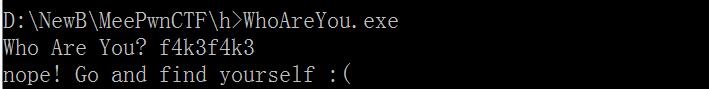In my opinion, the organizers play tricks that they modify the UPX compress algorithm’s implementation on their binary.

I also try to find OEP in x64dbg and then use Scylla to dump and rebuild the binary. But it seems that there exists anti-debug technique in the binary and the dumpped binary also gives me `f4k3f4k3`.

Why did I fail?

## Success

I failed to pass the challenge during the game. I have an idea that if I can dynamically follow the logic stream and dump the logic stream instructions out it may be effective. But I did not know how to realize it.

After seeing writeup by tonix0114, with CE (Cheat Engine) I make it finally even though my method seems not so graceful.

Based on what I did before, now I just need to extract instructions dynamically using CE: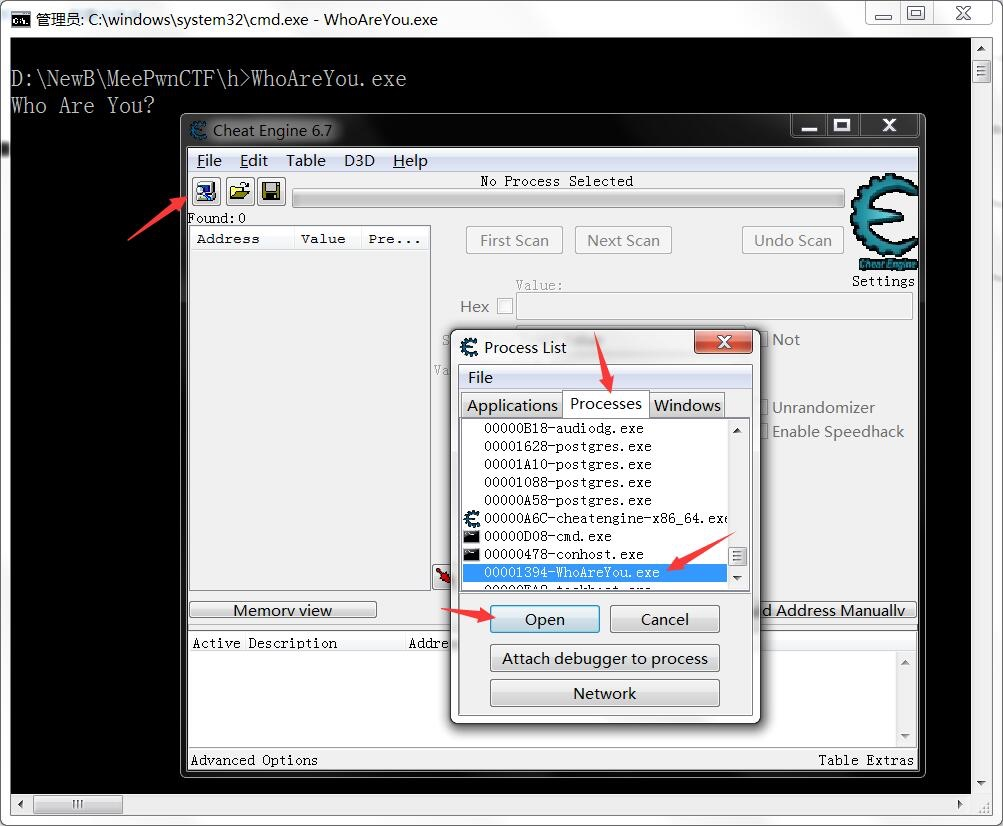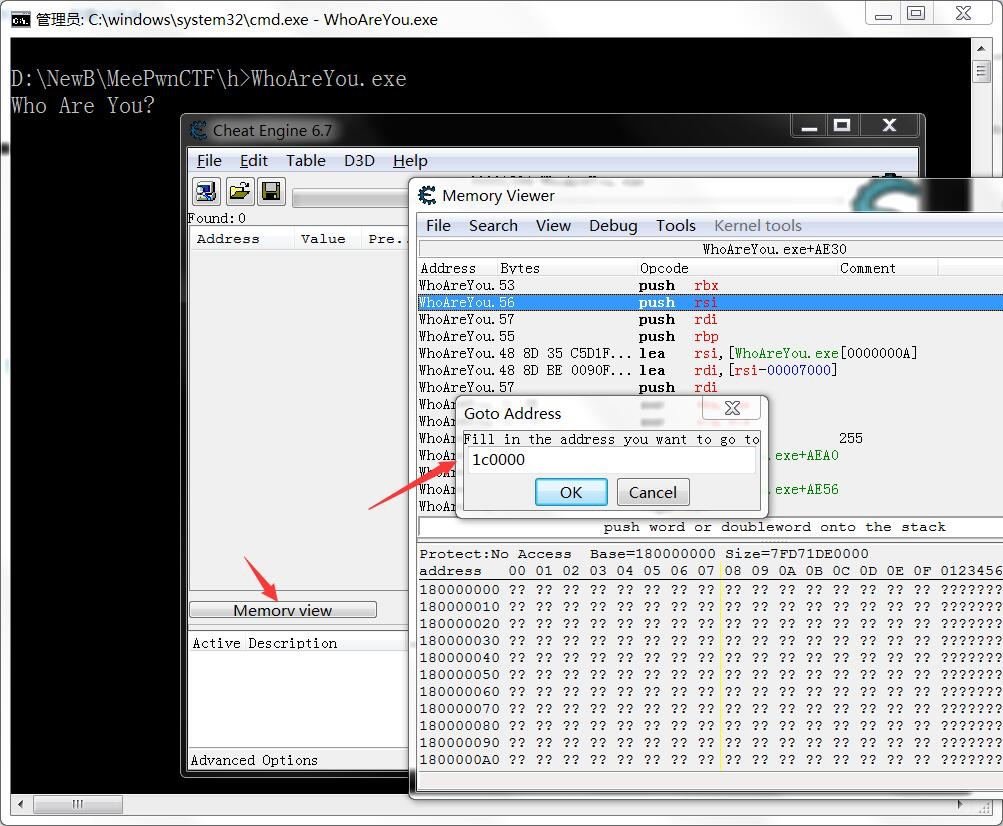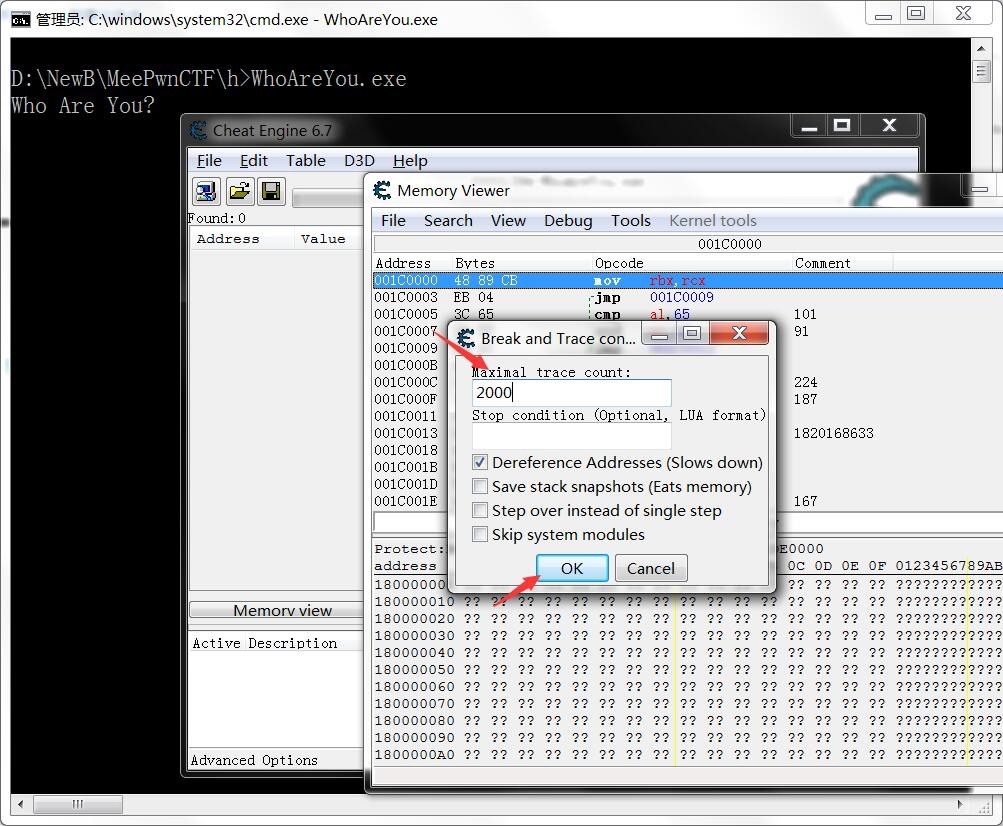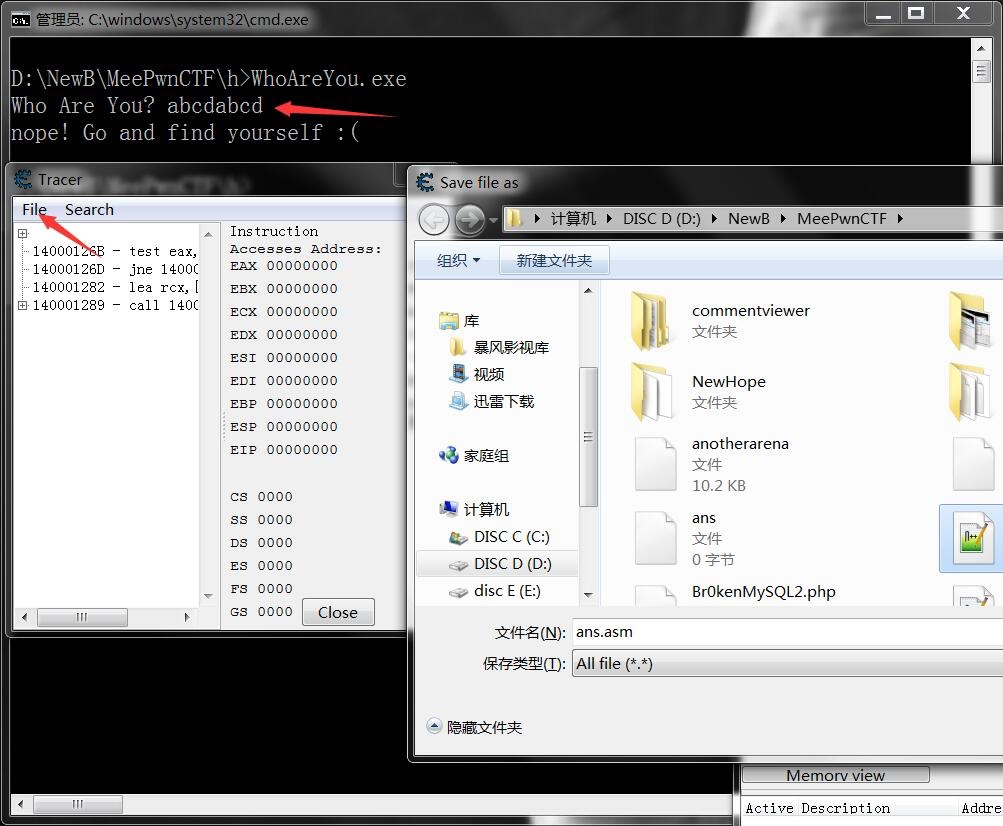Address `1C0000` are learned from x64dbg.

Notice that the assembly language instructions you get above should be adjusted in order to run on Linux. So you should use something like Sublime Text and Regex to modify them.

Then use `tac` and apply `decryption.py` on what you get.

After compilation, now use GDB to run it: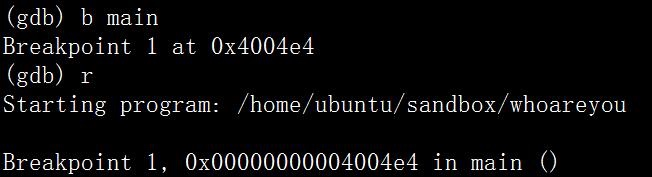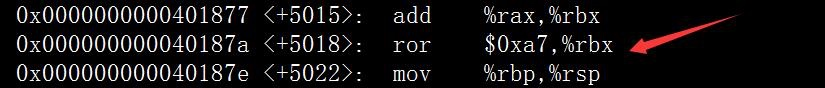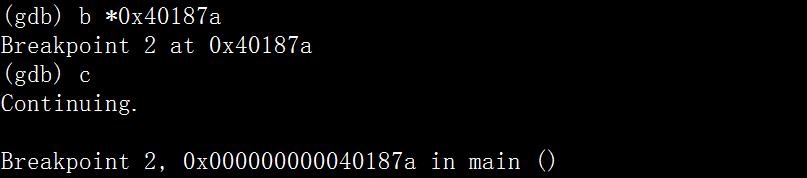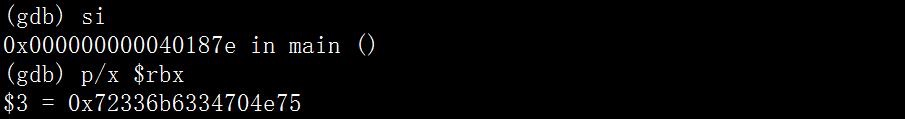Finally you get: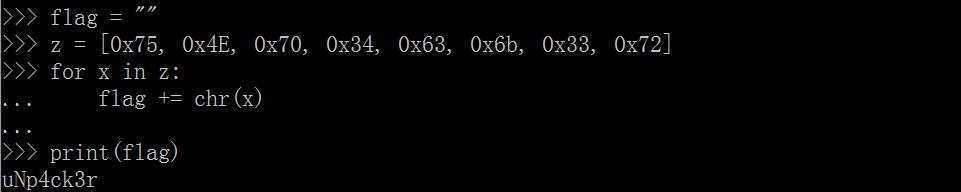## Other Method

tonix0114 wrote a simple Python script to simulate the assembly language interpreter:

``````with open("instruction") as f:

def ROL(data, shift, size=32):
shift %= size
remains = data >> (size - shift)
body = (data << shift) - (remains << size )
return (body + remains)

def ROR(data, shift, size=32):
shift %= size
body = data >> shift
remains = (data << (size - shift)) - (body << size)
return (body + remains)

"""
enc
-> mov eax, 0x123123123
enc[::-1]
-> mov eax, 0x123123123
dec(enc[::-1])
-> mov eax, 0x123123123
-> sub ebx, eax
"""
calc = []
for i in range(len(data)):
ins, op_str = data[i].split(" - ")[-1].split(" ")
if ins == "mov":
temp = calc.pop()
calc.append(ins + " " + op_str)
calc.append(temp)
else:
calc.append(ins + " " + op_str)

rax = 0
rbx = 0x6BA8F103D6E0FF17
check = " & 0xffffffffffffffff"

for i in range(0, len(calc)):
ins, op_str = calc[i].split(" ")
op1, op2 = op_str.replace("\n", "").split(",")
# minus
if op2 == "-":
op2 = hex(int(op2,16) & 0xff).replace("0x","")
# mov hex
if op2 not in ["rax", "rbx"]:
op2 = "0x" + op2

if ins == "mov":
exec(op1 +" = "+ op2 + check)
elif ins == "sub":
exec(op1 + " = (" + op1 + " + " + op2 + ")" + check)
exec(op1 + " = (" + op1 + " - " + op2 + ")" + check)
elif ins == "xor":
exec(op1 + " = (" + op1 + " ^ "  + op2 + ")" + check)
elif ins == "ror":
exec(op1 + " = " + "ROL(" + op1 + "," + op2 +  ", 64)" + check)
elif ins == "rol":
exec(op1 + " = " + "ROR(" + op1 + "," + op2 +  ", 64)" + check)
else:
print ins

flag = ""
while rbx:
flag += chr(rbx & 0xff)
rbx >>= 8
print flag
``````